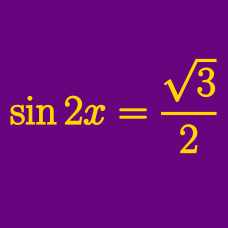Geometry

# Trigonometric Equations - Factoring

In the domain $$[0, 2 \pi ]$$, how many solutions are there to

$6 \cos^2 \theta - 10 \cos \theta + 3 \sin^2 \theta = 0 ?$

Which of the following are the solutions to the equation

$6 \tan^2 \theta - 17 \tan \theta + 2 \sec^2 \theta = 0 ?$

If $$2\pi \leq x < 4\pi$$, the sum of all the $$x$$'s that satisfy $\tan x+ \frac{1}{\tan x}-2=0$ can be expressed as $$a\pi$$. What is the value of $$2a?$$

The two roots of the quadratic equation $2x^2-x+k=0$ are $$\sin \theta$$ and $$\cos \theta$$. What is $16(\sin^3 \theta+\cos^3 \theta)?$

In the domain $$[0^\circ , 1000^\circ ]$$, how many solutions are there to $$4 \sin^2 \theta - 3 \sin \theta - 1 = 0$$?

×

Problem Loading...

Note Loading...

Set Loading...